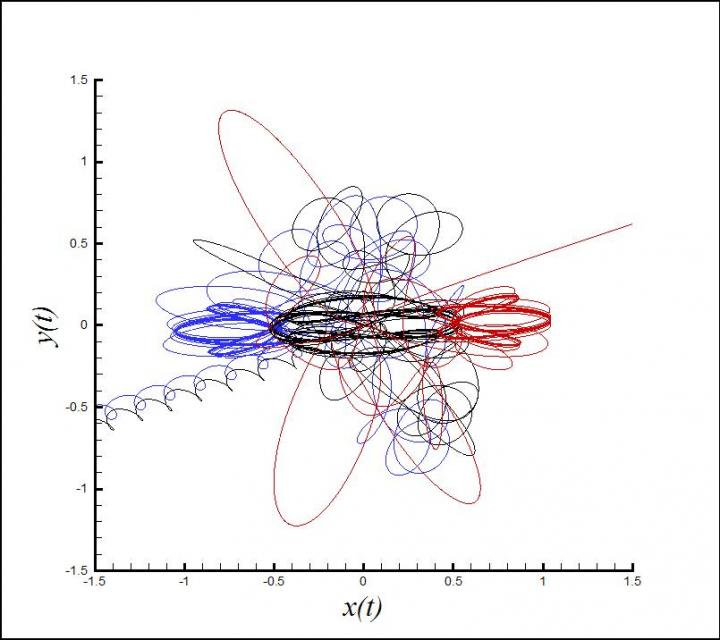News Release

# Some newly discovered periodic orbits of Newton's 3-body problem are unstable

Peer-Reviewed Publication

Science China Pressimage: This image depicts the orbits of three bodies in BUTTERFLY-I. view more  Credit: ©Science China Press

Isaac Newton's three-body problem, which examines how three bodies affected by gravitation will orbit around each other, is well known in nonlinear dynamics and applied mathematics. In his book "Philosophiæ Naturalis Principia Mathematica," published more than three centuries ago, Newton used the theory to describe the Moon's orbit around the Earth in light of the gravitational forces being exerted by the Moon, Earth and the sun.

Since then, scientists have strived to describe more complex orbital patterns involving three bodies in space.

In 2013, physicists Milovan Šuvakov and Veljko Dmitrašinović at the Institute of Physics in Belgrade reported: "We present the results of a numerical search for periodic orbits of three equal masses moving in a plane under the influence of Newtonian gravity, with zero angular momentum."

"A topological method is used to classify periodic three-body orbits into families, which fall into four classes, with all three previously known families belonging to one class," the two physicists added. "The classes are defined by the orbits geometric and algebraic symmetries. In each class we present a few orbits initial conditions, 15 in all; 13 of these correspond to distinct orbits."

The scientists, based at the University of Belgrade in Serbia, stated that their solutions were "either stable or marginally unstable, as otherwise they probably would not have been found."

Yet now, two researchers at Shanghai Jiao Tong University, in the eastern Chinese coastal city of Shanghai, report that at least 7 of these newly discovered families of orbits are unstable. In a new study published in the Beijing-based journal SCIENCE CHINA – Physics, Mechanics & Astronomy, Li Xiaoming and Liao Shijun state: "It is found that seven among these fifteen orbits greatly depart from the periodic ones within a long enough interval of time, and are thus most possibly unstable at least."

The three-body problem is prominent in nonlinear dynamics and applied mathematics, mainly because it is related to Isaac Newton, who took the first steps in the definition and study of the problem in his 1687 book "Principia."

The solution to the problem was considered very important and challenging at the end of the 19th century. In 1887, Oscar II, King of Sweden, established a prize for anyone who could find the solution. The prize was finally awarded to mathematician Henri Poincaré, who first found that there is no general analytical solution given by algebraic expressions and integrals for the three-body problem.

In general, orbits described by the three-body problem are non-periodic, i.e. chaotic, and are rather sensitive to initial conditions. According to the theory of chaos, uncertainty in initial conditions enlarges exponentially for chaotic dynamic systems. Therefore, it is rather difficult to gain reliable convergent numerical simulations of chaotic orbits of three-body systems across a long interval of time. Due to this, it is also difficult to find periodic orbits of three-body systems by means of numerical methods.

Liao Shijun at Shanghai Jiaotong University in China has developed a "Clean Numerical Simulation" (CNS) approach for reliable simulations of chaotic dynamic systems. Based on the arbitrary-order Taylor Series Method (TSM) and arbitrary digit precision of data with a procedure of solution verification, the CNS can reduce the numerical noise so greatly that reliable simulations of chaotic dynamic systems can be obtained in a rather long interval of time.

For example, Professor Liao and Dr. Wang used the supercomputer TH-A1 (at Tian-Jian, China) and applied the CNS with the 3500th-order Taylor series and 4180 digit precision data to gain, for the first time, a reliable convergent chaotic solution of Lorenz equation in a very long but finite interval [0,10000], whose correction is further validated using the 3600th-order Taylor series method and 4515 digit precision data (doi: 10.1007/s11433-013-5375-z). Such a reliable chaotic solution of Lorenz equation in a long interval of time has never been reported before. This indicates the validity and potential of the CNS for chaotic dynamic systems.

In the new study, Li and Liao used the CNS with the high-enough Taylor series method to stringently check the 13 new families of periodic orbits discovered by Milovan Šuvakov and Veljko Dmitrašinović, but found that seven of these families greatly depart from periodic orbits across a long interval of time, and are thus most possibly unstable. These results are helpful to deepening understanding of three-body problems.

###

See the article: Li Xiaoming, Liao Shijun. On the stability of the three classes of Newtonian three-body planar periodic orbits. SCIENCE CHINA Physics, Mechanics & Astronomy, 2014, 57(11): 2121-2126.